# Analog Transmission

To send the digital data over an analog media, it needs to be converted into analog signal.There can be two cases according to data formatting.

Bandpass:The filters are used to filter and pass frequencies of interest. A bandpass is a band of frequencies which can pass the filter.

Low-pass: Low-pass is a filter that passes low frequencies signals.

When digital data is converted into a bandpass analog signal, it is called digital-to-analog conversion. When low-pass analog signal is converted into bandpass analog signal, it is called analog-to-analog conversion.

## Digital-to-Analog Conversion

When data from one computer is sent to another via some analog carrier, it is first converted into analog signals. Analog signals are modified to reflect digital data.

An analog signal is characterized by its amplitude, frequency, and phase. There are three kinds of digital-to-analog conversions:

• Amplitude Shift Keying

In this conversion technique, the amplitude of analog carrier signal is modified to reflect binary data.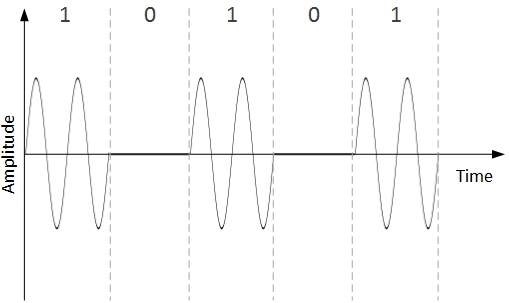When binary data represents digit 1, the amplitude is held; otherwise it is set to 0. Both frequency and phase remain same as in the original carrier signal.

• Frequency Shift Keying

In this conversion technique, the frequency of the analog carrier signal is modified to reflect binary data.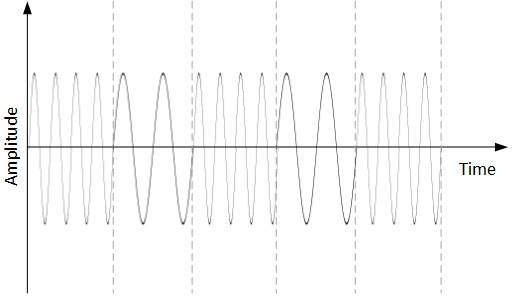This technique uses two frequencies, f1 and f2. One of them, for example f1, is chosen to represent binary digit 1 and the other one is used to represent binary digit 0. Both amplitude and phase of the carrier wave are kept intact.

• Phase Shift Keying

In this conversion scheme, the phase of the original carrier signal is altered to reflect the binary data.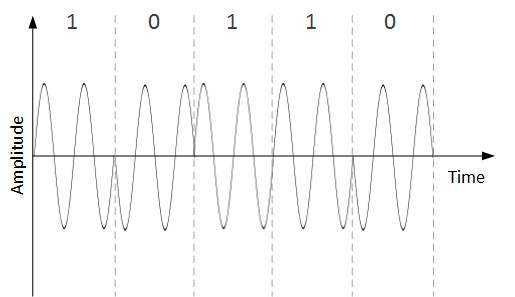When a new binary symbol is encountered, the phase of the signal is altered. Amplitude and frequency of the original carrier signal is kept intact.

QPSK alters the phase to reflect two binary digits at once. This is done in two different phases. The main stream of binary data is divided equally into two sub-streams. The serial data is converted in to parallel in both sub-streams and then each stream is converted to digital signal using NRZ technique. Later, both the digital signals are merged together.

## Analog-to-Analog Conversion

Analog signals are modified to represent analog data. This conversion is also known as Analog Modulation. Analog modulation is required when bandpass is used. Analog to analog conversion can be done in three ways:• Amplitude Modulation

In this modulation, the amplitude of the carrier signal is modified to reflect the analog data.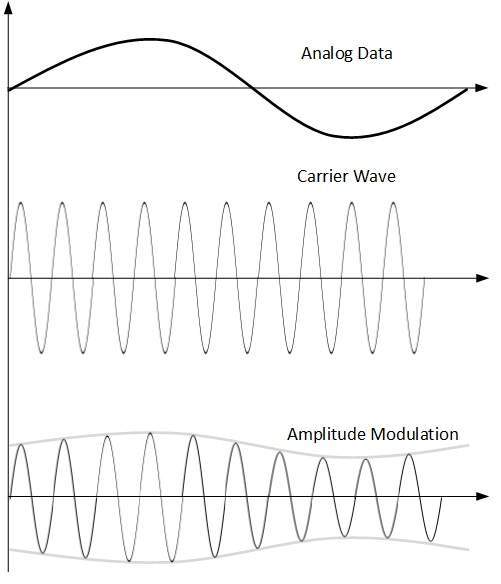Amplitude modulation is implemented by means of a multiplier. The amplitude of modulating signal (analog data) is multiplied by the amplitude of carrier frequency, which then reflects analog data.

The frequency and phase of carrier signal remain unchanged.

• Frequency Modulation

In this modulation technique, the frequency of the carrier signal is modified to reflect the change in the voltage levels of the modulating signal (analog data).The amplitude and phase of the carrier signal are not altered.

• Phase Modulation

In the modulation technique, the phase of carrier signal is modulated in order to reflect the change in voltage (amplitude) of analog data signal.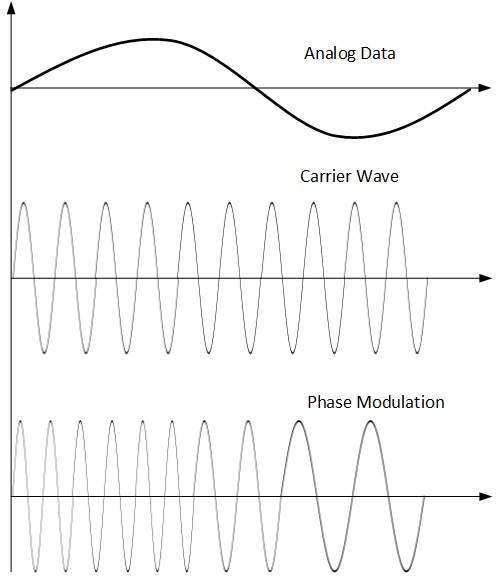Phase modulation is practically similar to Frequency Modulation, but in Phase modulation frequency of the carrier signal is not increased. Frequency of carrier is signal is changed (made dense and sparse) to reflect voltage change in the amplitude of modulating signal.